# OpenMP¶

## Data locality effects on scalability¶

Some investigation have been started on OpenMP-Performance and Data Locality:
• Simple application, which works on a single array:
• With Data Locality: source:/branches/cdo-openmp/test/openmp-test.c@1159
• Without Data Locality: source:/branches/cdo-openmp/test/openmp-test.c@1160 (see diff)
• Tests for 4, 8 and 16 thread and array size from 10000000 to 60000000
• GCC 4.4.3 on GNU/Linux x86_64

Here is, what came out on snow: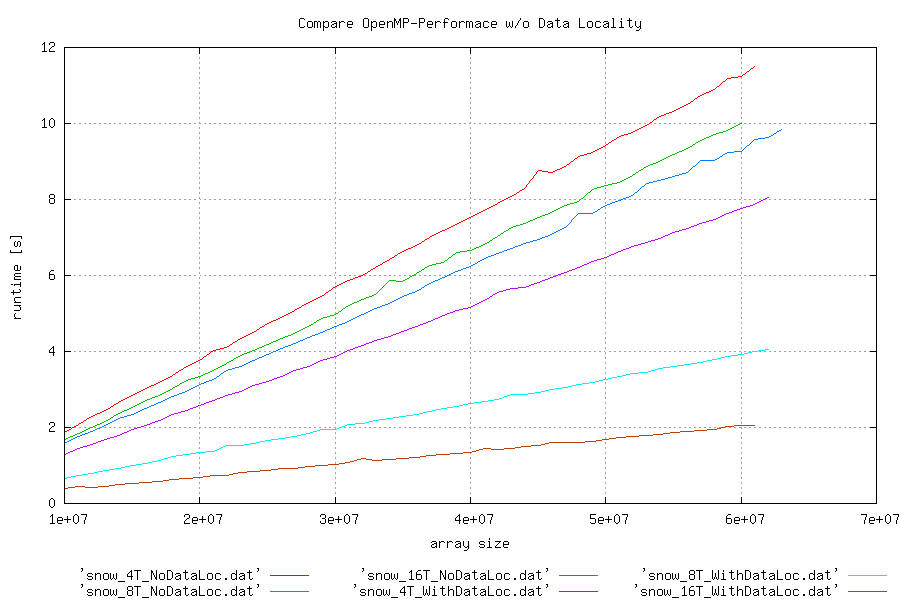## Comparing different kinds in initialization¶

In the above example, a performace consuming initialization has been used:

``````  array[i] = tan((double) (ompthID+i+0.5)/exp(-(double)ompthID));
``````
This should be replaced by zero values and lead to the same scalability effects, but reality seems to be a bit different:

• run script: r1246 (source:/branches/cdo-openmp/test/openmpCmp.sh@1246)
• makefile: source:/branches/cdo-openmp/test/Makefile@1244
• test code for init with zero: r1244 (source:/branches/cdo-openmp/test/openmp-test.c@1244)
• test code for init with math functione: r1245 (source:/branches/cdo-openmp/test/openmp-test.c@1245)
• if you are not logged in, please go to the very end of the page, where the source is displayed

Parallel Run of the binary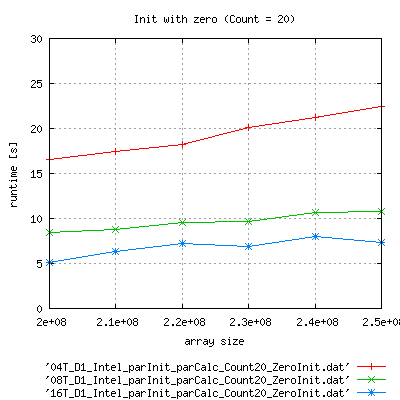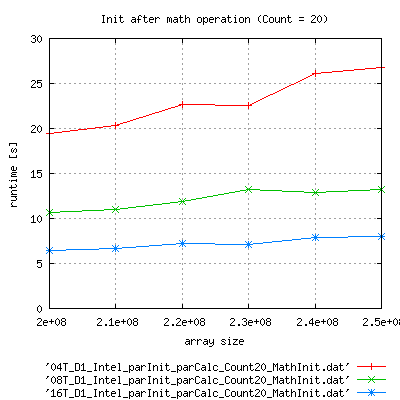Serial Run of the binary: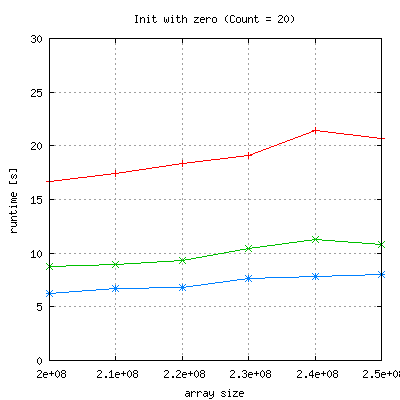Apart from the fact, that runtime with complex init is much larger than expected, is seems to scale better that with zero initialization.

## Compiler comparison: Intel vs. PGI vs. Sun vs GCC¶

The above code (init with zero) has been run 4 times with each compiler to investigate in the distribution of measurements. PGI (10.4), Suncc (SunStudio12) and gcc (4.4.3) are scaling very well, Intel (11.0.074) does not, but has a significant lower runtime: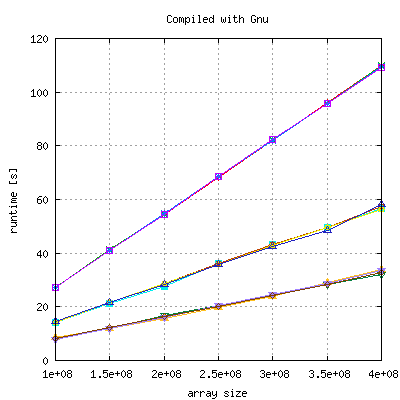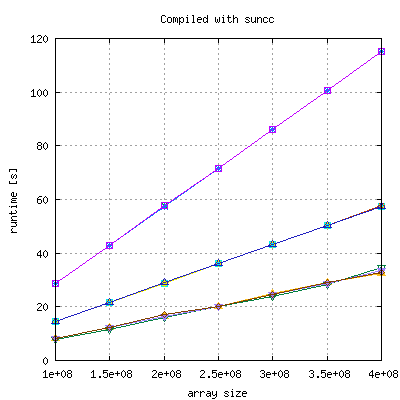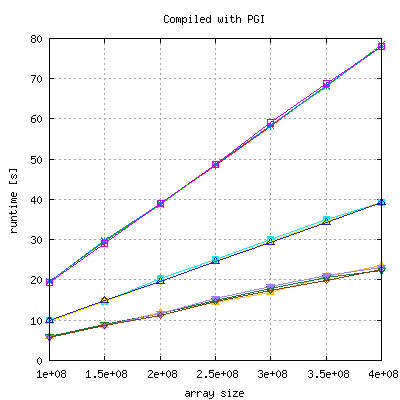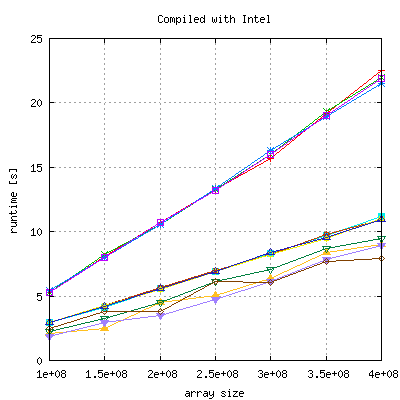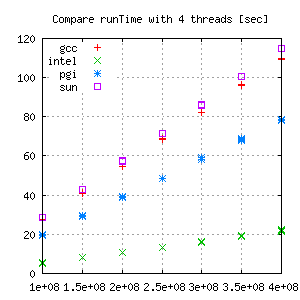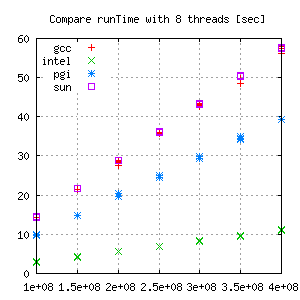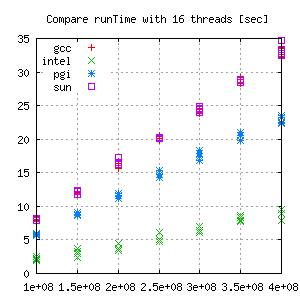This shows different error ranges for each compiler/gridsize. Looking closer the absolute error, there seems to be almost no trend.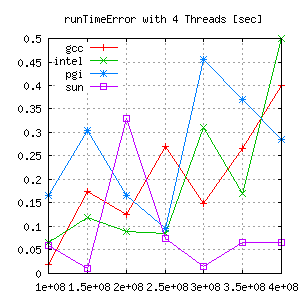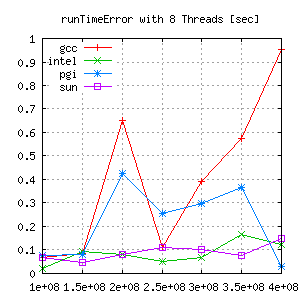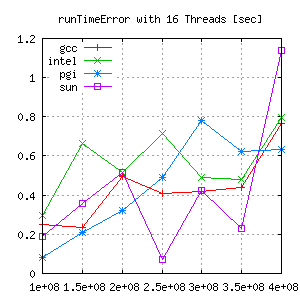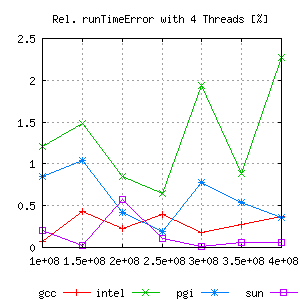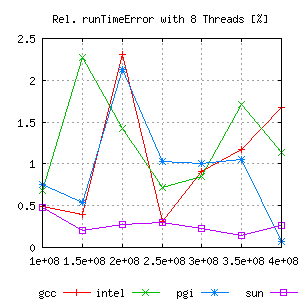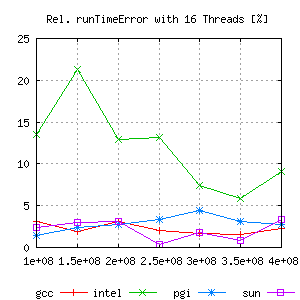Hence, for measuring scalability effects, the Intel Compiler does not seem to be the best tool. Gcc and even more suncc are better choices for these kind of tests.

## Getting real: Smaller grid size and complex algorithms¶

For this issue another version of the testing file is used (here or from repository). Basically the times of computing the math functions for each gridpoint is increased from 20 to 200 to keep the runtime on a level, where the thread overhead is negligible. The binary is run multiple times with different gridsizes. What came out is this: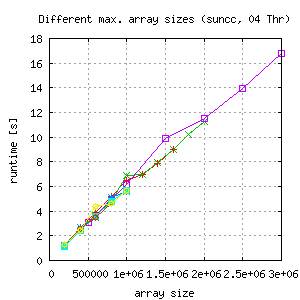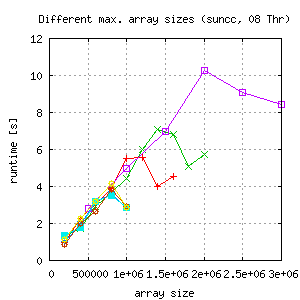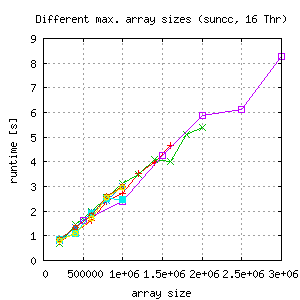• Runtime of the 16 thread run is only the half of the runtime with 4 threads, so scalability is lost.
• For 8 threads runtime decreases for larger grids depending on maximal array size of the last run of each test. The weird thing about this behaviour is, that each datapoint represents a separate run of the binary with the ame number of threads, same array portion for each thread because of the static schedule.
• Measurements with suncc compiled binaries, but gcc gives similar results.

A possible explanation could be the fact that this is an 8 socket machine (with quad core Opterons). A different scalability might be caused by this, but it should not do be responsible for the any interconnection of separate runs. This Paper suggests, that there are some special properties of trigonometric functions as well as `exp` or `log`, which might cause unexpected performance. I replaces the original computation

```
for (c = 0; c < COUNT; c++ )
{
array[i] = atan(array[i])*log((double)ompthID + 0.5);
}```
with this
```    for (c = 0; c < COUNT; c++ )
{
if ( 0 == c%2 )
{
val = sqrt(sqrt(val));
}
else
{
val = val*val*3.141529615243615234;
}
if ( 500 < val )
{
val = 0.500001*val;
}
}
```

With this change and a much larger loop count, the runtime (gcc and suncc) looks like this (4, 8 and 16 threads in one image):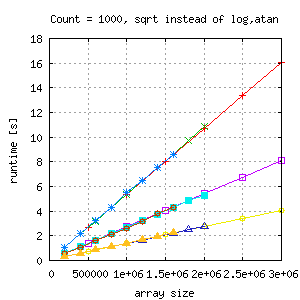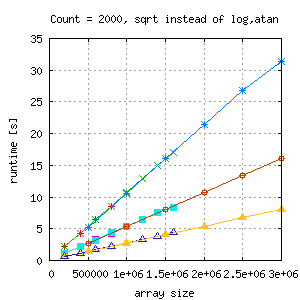Scalability looks perfect and measurements are independent. OpenMP scalability seems to depend not only on the general structure of algorithms but also on their constituent parts like mathematical functions. Just for making sure, that the results are repeatable, I ran the same binary the next morning: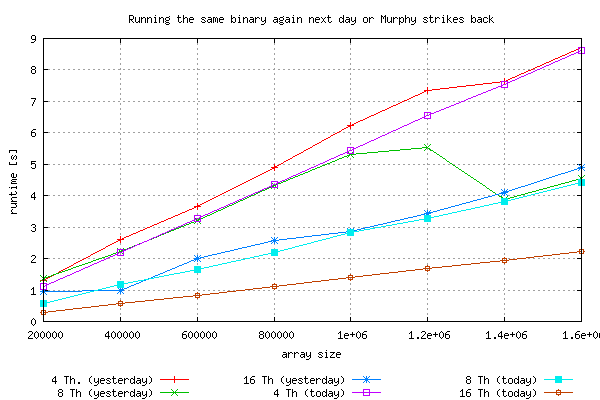The strange behaviour simply disappeared! Thanks Murph ...

## Scalability on multidimensional arrays¶

Memory is linearly aligned, so dimension issues are not expected. As shown in the picture below, the runtime scales with number of threads and with the memory usage.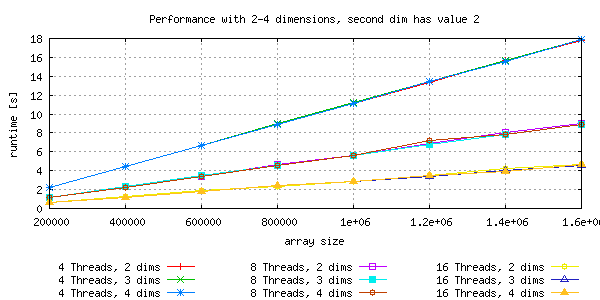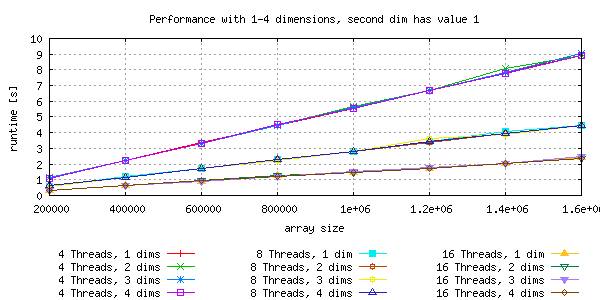### Increase dimensions up to reasonable number for levels and variables¶

Depending in how large each dimension is, it can be of high impact, which dimension is processed in parallel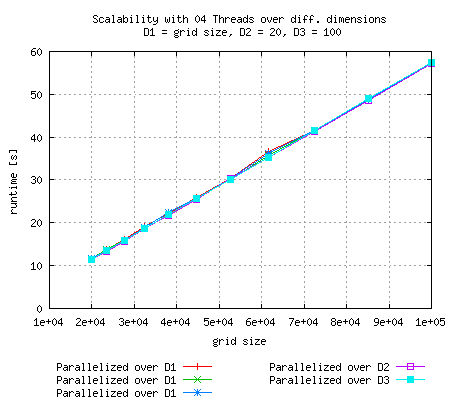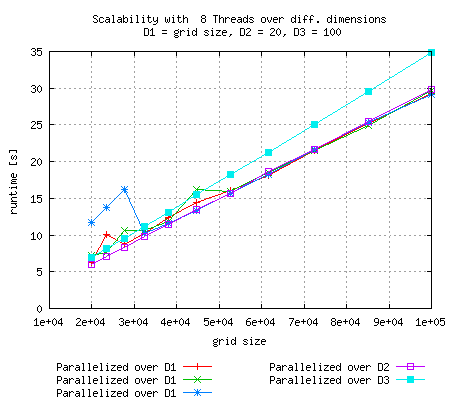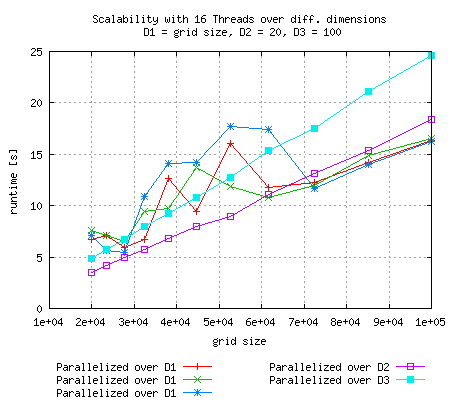Measurements with parallel for loop over the largest dimension have been repeated because of their non-linearity. In this case (D2 = 20, D3 = 100) doing the loop over D2 seems to be the best solution. It looks pretty linear and runtime is slower that parallalization over D3 and comparable to the parallel run of D1.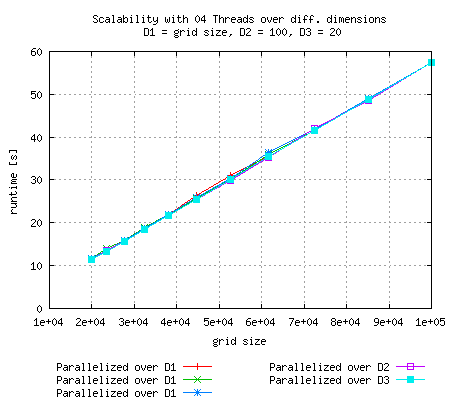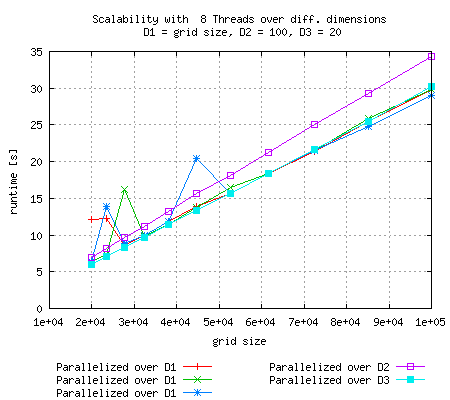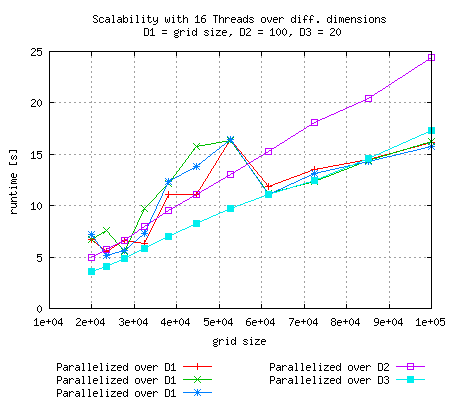Just like in the above experiment, runtime goes up for 16 threads and relatively small grid size and scaling is optimal if the smallest dimension is parallelized

=====================================================

## Testfile¶

``````#if defined (_OPENMP)
#  include <omp.h>
#endif

#include <stdlib.h>
#include <stdio.h>
#include <math.h>
#include "field.h"

#define WFORMATSTRING "LOG|gridID %d|thrID %d|W: %.2f\n"
#define RFORMATSTRING "LOG|gridID %d|thrID %d|R: %.2f\n"

#define DIMENSIONS 1
#define GRIDSIZE   10000001
#define LEVELS     1
#define VARS       1
#define TSTEPS     1
#ifdef  SINIT                   /* serial initialization */
#define PINIT      0
#else
#define PINIT      1            /* parallel initialization */
#endif
#if     SCALC                   /* serial calculation */
#define    PCALC      0
#else
#define    PCALC      1            /* parallel calculation */
#endif
#define COUNT      20
typedef struct
{
double *array;
} memory_t;

/*-----------------------------------------------------------------------------
* vector initialization for multiple dimensions
*-----------------------------------------------------------------------------*/
inline
static
void
initVec ( double *array, int size)
{
int i;
int ompthID;

#if defined (_OPENMP)
#pragma omp parallel for schedule(static) private(ompthID) if (PINIT)
#endif
for ( i = 0; i < size; i++ )
{
#if defined (_OPENMP)
#else
ompthID = 0;
#endif

array[i] = 0.0;

/*  array[i] = atan(array[i])*log((double)ompthID + 0.5);
*/

#if defined (OUT)
printf(WFORMATSTRING, (int)i, ompthID, array[i]);
#endif
}
return ;
}        /* -----  end of function vecRead  ----- */
inline
static
void
initVec2D ( double **array, int size0, int size1 )
{
int i;

for ( i = 0; i < size1; i++ )
initVec(array[i], size0);

return ;
}
inline
static
void
initVec3D ( double ***array, int size0, int size1, int size2 )
{
int i;

for ( i = 0; i < size2; i++ )
initVec2D(array[i], size0, size1);

return ;
}

inline
static
void
initVec4D ( double ****array, int size0, int size1, int size2, int size3 )
{
int i;

for ( i = 0; i < size3; i++ )
initVec3D(array[i], size0, size1, size2);

return ;
}

/*-----------------------------------------------------------------------------
*  calculation on multidimesional array
*-----------------------------------------------------------------------------*/
inline
static
void
calcVec ( double *array, int size )
{
int i,c;
int ompthID;

#if defined (_OPENMP)
#pragma omp parallel for schedule(static) private(ompthID,c) if (PCALC)
#endif
for ( i = 0; i < size; i++ )
{
#if defined (_OPENMP)
#else
ompthID = 0;
#endif

for (c = 0; c < COUNT; c++ )
array[i] = atan(array[i])*log((double)ompthID + 0.5);
/*
array[i] = atan(array[i])*log((double)ompthID + 0.5);
if ( 0 < array[i] && 10 > array[i])
array[i] = -array[i];
else
array[i] = 1.0;

array[i] = exp(array[i])*log((double)ompthID + 0.5);
if ( 0 > array[i] && -10 < array[i])
array[i] = -array[i];
else
array[i] = sin(exp(array[i]));
*/
#if defined (OUT)
printf(wFORMATSTRING, (int)i, ompthID, array[i]);
#endif
}
return ;
}        /* -----  end of function vecRead  ----- */

inline
static
void
calcVec2D ( double **array, int size0, int size1 )
{
int i;
for ( i = 0; i < size1; i++ )
calcVec(array[i], size0);

return ;
}

inline
static
void
calcVec3D (  double ***array, int size0, int size1, int size2 )
{
int i;

for ( i = 0; i < size2; i++ )
calcVec2D(array[i], size0, size1);

return ;
}

inline
static
void
calcVec4D (  double ****array, int size0, int size1, int size2, int size3 )
{
int i;

for ( i = 0; i < size3; i++ )
calcVec3D(array[i], size0, size1, size2);

return ;
}

/* static
* void
* memWrite ( memory_t *mem, int size, int chunk )
* {
*   int i;
*   int  j;
*   int ompthID;
*
* #if defined (_OPENMP)
* #pragma omp parallel for schedule(static) private(ompthID,j) shared(size,chunk)
* #endif
*   for ( i = 0; i < size; i++ )
*   {
* #if defined (_OPENMP)
* #else
*     ompthID = 0;
* #endif
*     for ( j = 0; j < chunk; j++ )
*     {
*       mem[ompthID].array[j] = tan((double) (ompthID+i+0.5)/exp(-(double)ompthID));
* #if defined (OUT)
*       printf(WFORMATSTRING, (int)i, ompthID, mem[ompthID].array[j]);
* #endif
*     }
*   }
* }
*
* static
* void
* memRead ( memory_t *mem, int size, int chunk )
* {
*   int i;
*   int  j;
*   int ompthID;
*
* #if defined (_OPENMP)
* #pragma omp parallel for schedule(static) private(ompthID,j) shared(size,chunk)
* #endif
*   for ( i = 0; i < size; i++ )
*   {
* #if defined (_OPENMP)
* #else
*     ompthID = 0;
* #endif
*     for ( j = 0; j < chunk; j++ )
*     {
*       mem[ompthID].array[j]  = atan(mem[ompthID].array[i])*log((double)ompthID + 0.5);
* #if defined (OUT)
*       printf(WFORMATSTRING, (int)i, ompthID, mem[ompthID].array[j]);
* #endif
*     }
*   }
* }
*
*/
int
main(int argc, char *argv[])
{
int ompthID;
int gridsize, dimensions, levels, vars, tsteps;
int tsID;
int i,j,k;
int nts,chunksize;
double *array;
double **array2D;
double ***array3D;
double ****array4D;
memory_t *mem = NULL;
/*   field_t **field; */

#if defined (_OPENMP)
#endif

gridsize   = ( argc > 1 ) ?  atoi(argv) : GRIDSIZE;
dimensions = ( argc > 2 ) ?  atoi(argv) : DIMENSIONS;
levels     = ( argc > 3 ) ?  atoi(argv) : LEVELS;
vars       = ( argc > 4 ) ?  atoi(argv) : VARS;
tsteps     = ( argc > 4 ) ?  atoi(argv) : TSTEPS;

#if defined (OUT)
printf ( "set dimesions to : %d\n", (int) dimensions );
printf ( "set gridsize to  : %d\n", (int) gridsize );
printf ( "set levels to    : %d\n", (int) levels );
printf ( "set vars to      : %d\n", (int) vars );
printf ( "set initmode to  : %d\n", (int) PINIT );
printf ( "set calcmode to  : %d\n", (int) PCALC );
#if defined (_OPENMP)
#endif
#endif
#if defined(EBUG)
return;
#endif
#if defined (MEM)
/*   chunksize = (int) gridsize/ompNumThreads + 1;
*
*   mem       = (memory_t *) malloc(ompNumThreads*sizeof(memory_t));
*   for ( i = 0; i < ompNumThreads; i++ )
*   {
*     mem[i].array = (double *) malloc(chunksize*sizeof(double));
*   }
*   memWrite(mem,gridsize, chunksize);
*
*
*/
#else
switch (dimensions)
{
case 1:
array = (double *) malloc(gridsize*sizeof(double));

initVec(array, gridsize);
calcVec(array, gridsize);

free(array);
break;
case 2:
array2D = (double **) malloc(levels*sizeof(double *));
for ( i = 0; i < levels; i++)
array2D[i] = (double *) malloc(gridsize*sizeof(double));

initVec2D(array2D, gridsize, levels);
calcVec2D(array2D, gridsize, levels);

for ( i = 0; i < levels; i++)
free(array2D[i]);
free(array2D);
break;
case 3:
array3D = (double ***) malloc(vars*sizeof(double **));
for ( i = 0; i < vars; i++)
{
array3D[i] = (double **) malloc(levels*sizeof(double *));
for ( j = 0; j < levels; j++)
array3D[i][j] = (double *) malloc(gridsize*sizeof(double));
}

initVec3D(array3D,gridsize,levels,vars);
calcVec3D(array3D,gridsize,levels,vars);

for ( i = 0; i < vars; i++)
{
for ( j = 0; j < levels; j++)
free(array3D[i][j]);
free(array3D[i]);
}
free(array3D);
break;
case 4:
array4D = (double ****) malloc(vars*sizeof(double ***));
for ( k = 0; k < tsteps; k++ )
{
array4D[k] = (double ***) malloc(vars*sizeof(double **));
for ( i = 0; i < vars; i++)
{
array4D[k][i] = (double **) malloc(levels*sizeof(double *));
for ( j = 0; j < levels; j++)
array4D[k][i][j] = (double *) malloc(gridsize*sizeof(double));
}
}

initVec4D(array4D,gridsize,levels,vars,tsteps);
calcVec4D(array4D,gridsize,levels,vars,tsteps);

for ( k = 0; k < tsteps; k++ )
{
for ( i = 0; i < vars; i++)
{
for ( j = 0; j < levels; j++)
free(array4D[k][i][j]);
free(array4D[k][i]);
}
free(array4D[k]);
}
free(array4D);
break;
default:

printf ("default case\n" );
}
#endif
return (0);
}``````